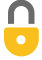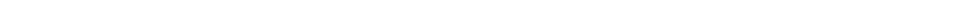Problem 34
A
a) $2.10 \times 10^{-3} \textrm{kgm}^2$
b) $0.0718 \textrm{Nm}$In order to watch this solution you need to have a subscription.By Dfirst05 on Fri, 2/13/2015 - 4:57 AM

On part B shouldn't the final answer be Applied Torque = 0.0598 Nm since you are adding a negative frictional torque?

By Mr. Dychko on Sat, 2/14/2015 - 12:13 AM

Hi Dfirst05, thanks for the question about negative signs with torque. Depending on your perspective, the video has a slight error. Let me explain. At 3:59, I mention that the frictional torque $\tau_{fr} = 0.005983 \textrm{ N m}$, positive. Technically, you are correct in saying the frictional torque should be negative since I took the angular acceleration due to friction to be negative. As a matter of personal preference, and as an obvious source of confusion for students in this case (sorry!), I just wanted the magnitude of the frictional torque, without caring about it's sign. The reason for this is that later, when I state the net torque, I wrote it as $\tau_{applied} - \tau_{fr} = I \alpha$, and take notice of the minus in this equation. That minus in the equation accounts for the direction. My personal preference is to write Net Force or Net Torque with pluses and minus in the equation to account for direction, and then always substitute magnitudes in for the values. Not everyone does it this way. The alternative is to write the net torque as $\tau_{applied} + \tau_{fr} = I \alpha$ and substitute a negative frictional torque. So the two ways to a correct answer are:

#### Option 1: put negatives in the net torque equation, and substitute magnitudes

This is the option used in the video. In this case $\tau_{applied} - \tau_{fr} = I \alpha$ and torques are always positive, no matter what, since their direction are accounted for in the equation.

#### Option 2: always put positives in the net torque equation, and substitute torques with their correct signs

This option is more common in textbooks. In this case $\tau_{applied} + \tau_{fr} = I \alpha$ and the frictional torque would be substituted as a negative number. With this approach you would be subtracting a negative frictional torque at 6:22 in the video since the final equation would be $\tau_{applied} = I \alpha - \tau_{fr}$

With either approach, the correct answer is $0.0718 \textrm{ N m}$. Both approaches for doing net torque (or net force) are common, and it's just important to recognize which approach is being used, and being consistent.

I hope this helps,
Mr. Dychko

Giancoli Answers, including solutions and videos, is copyright © 2009-2023 Shaun Dychko, Vancouver, BC, Canada. Giancoli Answers is not affiliated with the textbook publisher. Book covers, titles, and author names appear for reference purposes only and are the property of their respective owners. Giancoli Answers is your best source for the 7th and 6th edition Giancoli physics solutions.